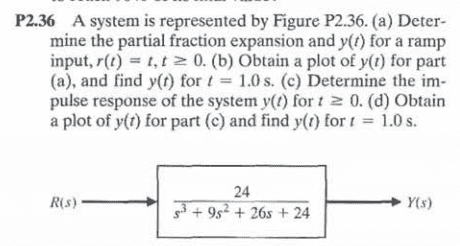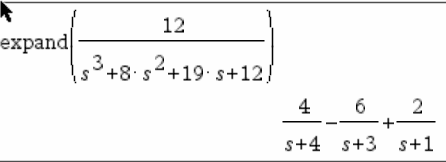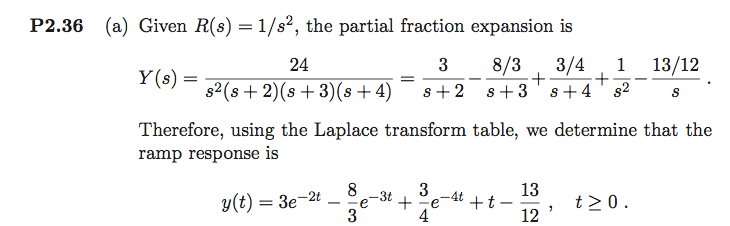# Help with partial fraction in control systems

Huumah
I'm having problem with task (a) in this problem

The questionMy attemptThe solutionWhy don't I get the same after I take the partial fraction using my calculator? And where does this R(s)=1/s^2 come from

Mentor
1/s2 is the Laplace transform of the driving signal R(s). The Laplace transform of a ramp r(t)=t is R(s) = 1/s2.

Remember that a transfer function F(s) is the Laplace domain equivalent of the ratio of the output signal to the input. So if the input is R(s) then:

Y(s) = R(s)*F(s)

The transfer function that you've wrapped in "expand" doesn't appear to correspond to the one given in the problem statement.

•1 person
Huumah
Thanks. I copied the problem from a pdf i found online to post here. But I guess they changed the numbers in the problem cause it's not the same numbers as in my book. So the solution manual doesn't show the correct answers to my book even though they are the same editon.

Staff Emeritus
•